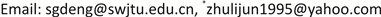﻿ 差分方程xn+1=xn/(p+xn-1)的动力学性质 Dynamics of the Difference Equation xn+1=xn/(p+xn-1)

Vol. 07  No. 11 ( 2018 ), Article ID: 27627 , 3 pages
10.12677/AAM.2018.711163

Dynamics of the Difference Equation ${x}_{n+1}={x}_{n}/\left(p+{x}_{n-1}\right)$

Shaogao Deng1, Lijun Zhu2*

1School of Mathematics, Southwest Jiaotong University, Chengdu Sichuan

2School of Mathematics and Information Science, North Minzu University, Yinchuan NingxiaReceived: Oct. 23rd, 2018; accepted: Nov. 13th, 2018; published: Nov. 20th, 2018ABSTRACT

This paper considers the difference equation ${x}_{n+1}={x}_{n}/\left(p+{x}_{n-1}\right)\left(p\ge 0,n\ge 2\right)$ with the initial values ${x}_{1}>0,{x}_{2}>0$ . The asymptotic stability of the positive solutions is proved under some assumptions.

Keywords:Difference Equation, Equilibrium Point, Asymptotic Stability1西南交通大学数学学院，四川 成都

2北方民族大学数学与信息科学学院，宁夏 银川1. 引言

${x}_{n+1}={x}_{n}/\left(p+{x}_{n-1}\right)$ (1.1)

${x}_{n+1}=f\left({x}_{n},{x}_{n-1}\right)\left(n\ge 2\right)$ (1.2)

${\lambda }^{2}-{f}_{x}\left(\beta ,\beta \right)\lambda -{f}_{y}\left(\beta ,\beta \right)=0$ (1.3)

2. 主要结果

1) 当 $p\ge 1$ 时，有唯一的平衡点 $\beta =0$ ，且是全局(渐近)稳定的；

2) 当 $0 时，有两个平衡点 $\beta =0$$\beta =1-p$ ，其中 $\beta =0$ 是不稳定的，而 $\beta =1-p$ 是(渐近)稳定的。

1) 当 $p\ge 1,n\ge 2$ 时， ${x}_{n+1}/{x}_{n}=1/\left(p+{x}_{n-1}\right)<1/p\le 1$

$\beta =0或\beta =1-p\left(当p>1时舍去\right)$

2) 当 $0 时，令 ${x}_{n}\equiv \beta$ ，可得：

$\beta =0或\beta =1-p>0$

$f\left(x,y\right)=x/\left(p+y\right)$ ，则：

${f}_{x}\left(x,y\right)=1/\left(p+y\right)$${f}_{y}\left(x,y\right)={-x/\left(p+y\right)}^{2}$

$\beta =0$ 时， ${f}_{x}\left(\beta ,\beta \right)=1/p$${f}_{y}\left(\beta ,\beta \right)=0$

${\lambda }_{1}=0,{\lambda }_{2}=1/p>1$

$\beta =1-p$ 时， ${f}_{x}\left(\beta ,\beta \right)=1$${f}_{y}\left(\beta ,\beta \right)=p-1$

$0<{\lambda }_{1}=\left(1-\sqrt{4p-3}\right)/2\le {\lambda }_{2}=\left(1+\sqrt{4p-3}\right)/2<1\left(当3/4\le p<1时\right)$

${‖{\lambda }_{1}‖}^{2}={‖{\lambda }_{2}‖}^{2}={\lambda }_{1}{\lambda }_{2}=1-p<1\left(当0

${x}_{3}=b/a,{x}_{4}=1/a,{x}_{5}=1/b,{x}_{6}=a/b,{x}_{7}=a,{x}_{8}=b\cdots \cdots$

Dynamics of the Difference Equation xn+1=xn/(p+xn-1)[J]. 应用数学进展, 2018, 07(11): 1402-1404. https://doi.org/10.12677/AAM.2018.711163

1. 1. 周义仓, 曹慧, 肖燕妮. 差分方程及其应用[M]. 北京: 科学出版社, 2014.

2. 2. Elaydi, S. (2005) An Introduction to Difference Equations. 3rd Edition, Springer-Verlag, New York.

3. 3. Amleh, A.M., Grove, E.A., Ladas, G. and Georgiou, D.A. (1999) On the Recursive Sequence . Journal of Mathematical Analysis and Applica-tions, 233, 790-798. https://doi.org/10.1006/jmaa.1999.6346

4. 4. 徐胜荣, 王希超, 周营营. 一类差分方程的稳定性研究[J]. 山东农业大学学报(自然科学版), 2013, 44(4):624-629.

5. 5. 韩彩虹, 李 略, 黄荣里. 差分方程 的动力学性质[J]. 广西师范大学学报(自然科学版), 2013, 31(1): 44-47.

6. NOTES

*通讯作者。